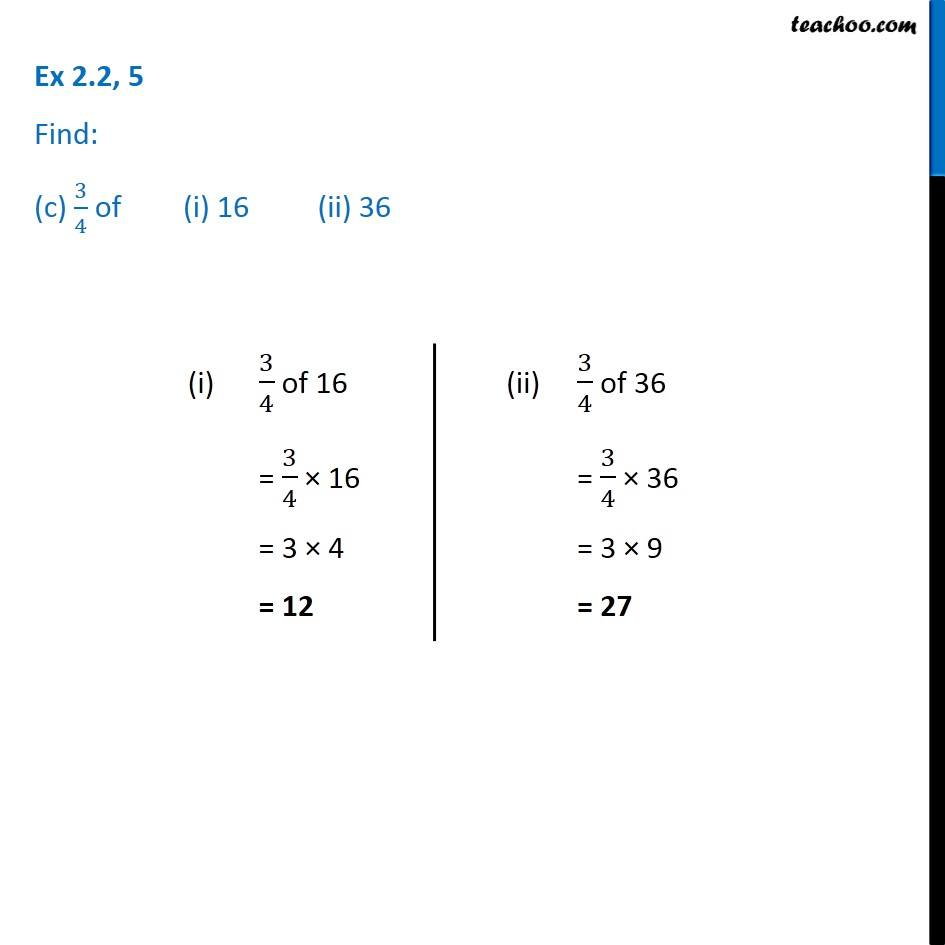1. Chapter 2 Class 7 Fractions and Decimals
2. Serial order wise
3. Ex 2.2

Transcript

Ex 2.2, 5 Find: (c) 3/4 of (i) 16 (ii) 36 (i) 3/4 of 16 = 3/4 × 16 = 3 × 4 = 12 (ii) 3/4 of 36 = 3/4 × 36 = 3 × 9 = 27